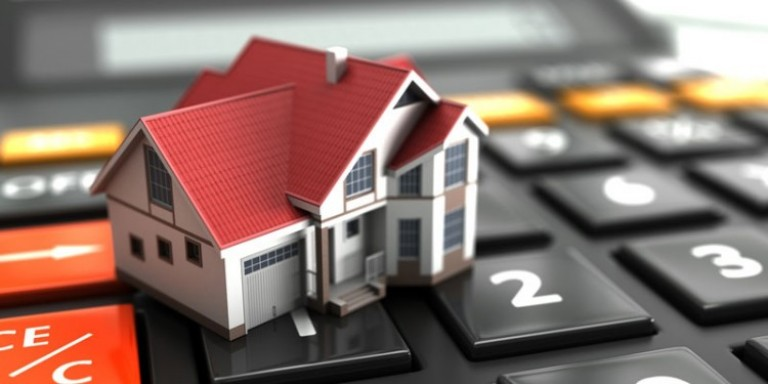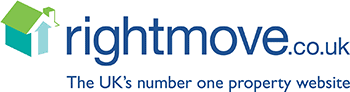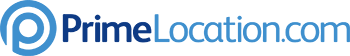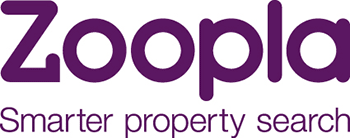# How is Buy to Let Affordablity Assessed

Buy to Let Mortgage affordability is calculated by ensuring that the rent payable is over the minimum mortgage that will be required to be paid (AND in most cases a minimum personal income requirement.)

Their are three variables to formula mortgage lenders use - the Rent/the Loan, the Multiplier and the Rate.

the Rent: is what rent can be achieved at the property. Often this value comes not from the Tenancy Agreement but what a valuer may think you can achieve.
or the Loan: how much you can borrow.

the Multiplier: is a percentage of which the Rent of the property must exceed. The bank does not just want the rent to cover the mortgage, with £0 left over. They may want the mortgage plus 20%, therefore 120% multiplier.

the Rate: is a interest rate the lender decides to stress test by. Sometimes a rate a lenders risk department decides but more often the lenders Standard Variable Rate (SVR).

The Two Buy to Let Affordability Formulas

You can use the formula in two ways:

Rent to Max Loan Formula
Given the Rent you can achieve what is the maximum loan a lender will offer:

theRent (divide) theMultiplier (divide) theRate (multiply) 12 months

Rent Required for Loan Formula
Given the Loan you want to borrow, what is the minimum rent a lender will require:

theLoan (divide) 12 months (multiply) theRate (multiply) theMultiplier

What MULTIPLIER and RATE do Lenders Use?

Each lenders risk department decides different rates - depending on there risk profile and how much market share they target for. The multipliers range from 110% to 150% and the Rates range from 5% to 6%

## Recent Blogs